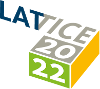#The 39th International Symposium on Lattice Field Theory (Lattice 2022)

Aug 8 – 13, 2022
Hörsaalzentrum Poppelsdorf
Europe/Berlin timezone

## Removing the $N\pi$ contamination from axial matrix elements at non-vanishing momentum

Aug 12, 2022, 4:40 PM
20m
CP1-HSZ/0.010 (CP1-HSZ) - HS2 (CP1-HSZ)

450
Show room on map

### Speakers

Mr Lorenzo Barca (Universität Regensburg) Lorenzo Barca (Universität Regensburg)

### Description

Excited state contamination is one of the most challenging sources of systematics to tackle in the determination of nucleon matrix elements and form factors. The signal-to-noise problem prevents one from considering large source-sink time separations. Instead, state-of-the-art analyses consider multi-state fits. Excited state contributions to the correlation functions are particularly significant in the axial channel. In this work, we confront the problem directly. Since the major source of contamination is understood to be related to pion production, we consider three-point correlators with a $N$ operator at the source and a $N\pi$ interpolating operator at the sink, which allows studies of $N \to N\pi$ matrix elements. After discussing the challenges that arise when using a two-particle interpolating operator, like the projection onto the proper irreducible representation and on the isospin components, we present solutions of the Generalised Eigenvalue Problem for a matrix of two-point functions
constructed using different bases of $N$ and $N\pi$ operators. We adopt the GEVP results to present improved $N \to N$ axial and pseudoscalar matrix elements, where we remove directly the contamination from $N\pi$ states, on a $m_\pi \approx 420$ MeV ensemble.

### Primary author

Mr Lorenzo Barca (Universität Regensburg)

### Co-authors

Prof. Gunnar Bali (Universität Regensburg) Dr Sara Collins (Universität Regensburg)